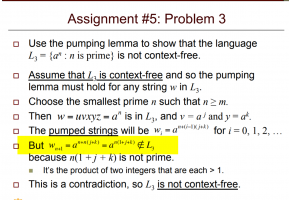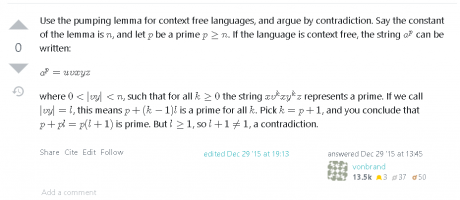# Why did we choose n+1 in this proof of pumping lemma for context free language? I can't understand what are we trying to show by choosing i=n+1

#### shivajikobardan

##### Junior MemberEven heresame thing is done, what is the reason behind choosing i= givenprimelength+1

Here is more detail.

#### blamocur

##### Senior Member
Choosing [imath]i = n+1[/imath] gets you a non-prime number [imath]n+n*(j+k) = (n+1)(j+k)[/imath]. But this is the length of a "pumped" string, which, according to the lemma, must be in [imath]L_3[/imath] and thus have a prime length. The resulting contradiction proves that a set of all strings of prime length is not a context-free language.